# Items We Have Available

Acquired in 2020:

24. These magnetic foam fraction circles are a fun way to learn about arithmetic, especially fractions, and encourage team work for young leaners. These were used in Math 4100 (Topics in Math Education) for our teacher candidates.  Credit: Professor Andrew Skelton.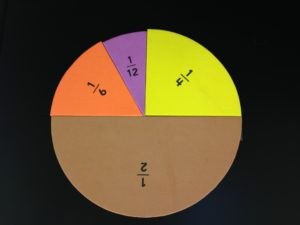23. Roll this Tumble 'N Teach Dice! Operations are on the outside and two dies are on the inside. These dies are a fun way to integrate arithmetic, randomization and physical activity for young leaners. These were used in Math 4100 (Topics in Math Education) for our teacher candidates.  Credit: Professor Andrew Skelton.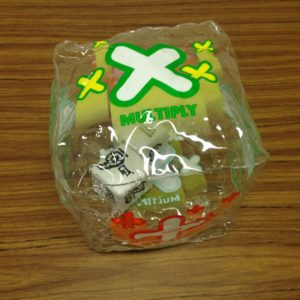22. Cuisenaire rods are tactile aids that explore arithmetic, especially fractions, for young leaners. These were used in Math 4100 (Topics in Math Education) for our teacher candidates.  Credit: Professor Andrew Skelton.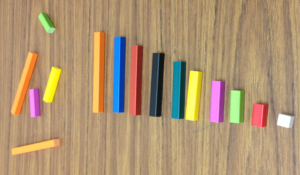21. Attribute math blocks are tactile aids that explore such as concepts as geometric shapes, patterns, arithmetic, Venn Diagrams and mathematical reasoning for young leaners. These were used in Math 4100 (Topics in Math Education) for our teacher candidates.  Credit: Professor Andrew Skelton.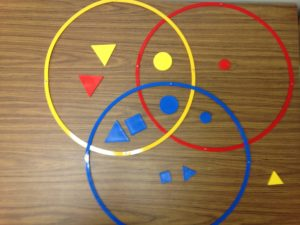Acquired in 2019:

20. This experiment creates standing waves in a string using a string vibrator. Both ends of the string are fixed, and nodes/antinodes are clearly visible. The vibrating string experiment was used at the CMS Math Summer Camp to demonstrate experimental math, mathematical modelling and vibrations.  Credit: Professor Hongmei Zhu.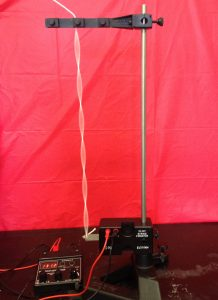19. These double length slinkies (152cm in length) aid in the visualization and generation of waves, and hence model vibrations and ideas related to it such as amplitude, frequency, period, oscillation, sinusoidal curves, etc. These slinkies were used at the CMS Math Summer Camp to demonstrate experimental math, mathematical modelling and vibrations. Credit: Professor Hongmei Zhu.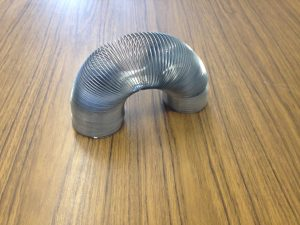18. Similar to the zoomtools (item 17 below), polydron frameworks are manipulative tools for building and exploring geometric shapes. The cube pictured below is constructed from polydron frameworks. These were used in Math 4100 (Topics in Mathematical Education) and Math 3052 (Exploring Geometries). Credit:  Professor Andrew Skelton and Professor Walter Whiteley.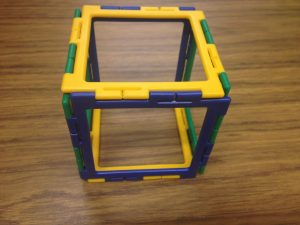17. Zometools are manipulatives that facilitate the visualization and construction of geometric shapes. These were used in Math 4100 (Topics in Mathematical Education). The blue and yellow cube like structure in the picture below was constructed from zometools. Credit:  Professor Andrew Skelton and Professor Walter Whiteley.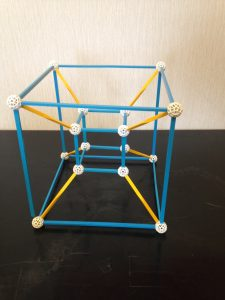16. This is a mirascope (15cm in diameter) which can be used to create a hologram.  The mirascope consists of two parabolic shaped mirrors facing each other. The top mirror has a hole in its centre. The actual frog is placed at the bottom of the mirror. Through reflections between the two mirrors, an image of the frog appears on top of the hole. Here is a schematic of how the mirascope works.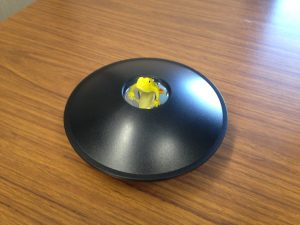15. This is a resonance experiment, which consists of Chladni plates (in the shape of a 24cm sided square, 24 cm diameter circle, 40cm long violin shape), a mechanical wave driver and a sine wave generator. The plate sits on top of the mechanical wave driver which creates vibrations that are controlled by the sine wave generator.  Sand is sprinkled evenly on top of the vibrating plate, and eventually the sand settles to stable nodes creating symmetric patterns, called Chladni figures, that can be predicted by the wave equation and elasticity theory. A video of this (keep volume down!). This is a classic experiment first made famous by Ernest Chladni (1756 - 1827).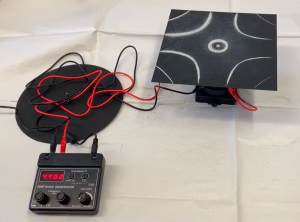The appearance of the Chladni figure depends on the shape of the plate and the frequency of the sine wave generator.  The mathematical theory behind Chladni figures was derived by Sophie German (1776 - 1831). This experiment was used at the Fields Institute "A Celebration of Women Mathematicians" (May 13, 2019) and during our CMS summer math camps. The experiment can be simplified to the one-dimensional case by replacing the plates with a string.  Credit: Allysa Lumley, Yohana Solomon and Professor Hongmei Zhu.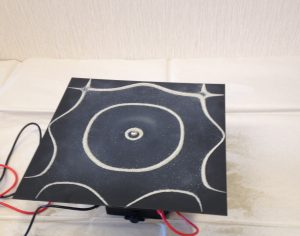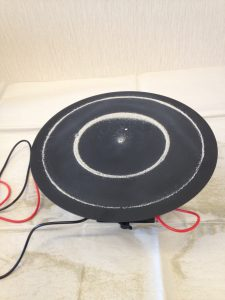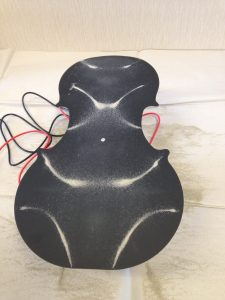14. A disc and circle of the same size and weight are racing down a ramp. Which one always wins the race? This demonstration was used in Math 2015 (Applied Multivariate and Vector Calculus) to motivate moments of inertia. It was also used in Math 3010 (Vector Integral Calculus). See the video. Credit:  Professor Andrew Skelton.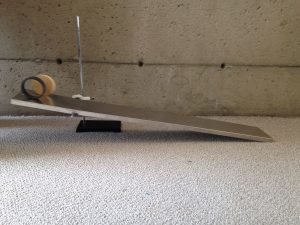13. This is a Sphero Bolt, which is an app-enabled robot with a 7.3cm diameter. It was used in Math 2015 (Applied Multivariate and Vector Calculus), Math 3052 (Exploring Geometries), and MATH 4100 (Topics in Mathematical Education). Examples of mathematical concepts that the Sphero Bolt will help students learn include graphing, geometry, parameterizations and programming.  It was also used for a student math project about finding the optimal path on the plane given obstacles along the path, which required programming into the sphero bolt a shortest path algorithm known as Jarvis’ March and explored mathematical concepts such as convex hulls and optimization.   Credit:  Professor Andrew Skelton.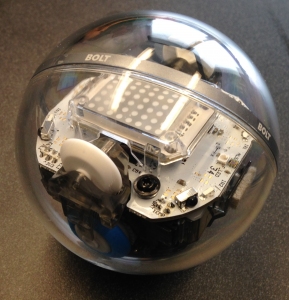Acquired in 2018:

12. This is a rotary servo base unit (approximately 15cmX15cmX18cm) from Quanser, which steers the dynamics of mounted or attached appendages such as a pendulum, flexible link or joint, and beam. The software this rotary unit uses is MathWork's Simulink. It was used in Math 3052 (Exploring Geometries) and MATH 4650 (Feedback Control Systems).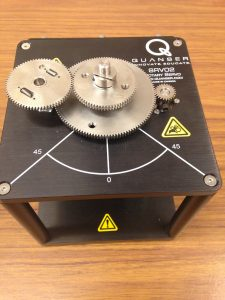11. This is a ball on a beam (length is 42.55cm) with one end of the beam fixed and the other end attached to a rotary servo base unit (approximately 15cmX15cmX18cm) that controls the movement of the ball and beam. This apparatus is from Quanser.  To hold the ball at equilibrium on the beam requires stability theory and feedback control. The software this rotary unit uses is MathWork's Simulink. It was used in Math 3052 (Exploring Geometries) and MATH 4650 (Feedback Control Systems).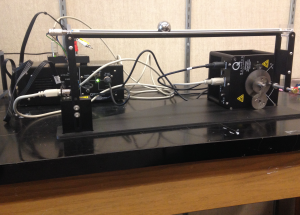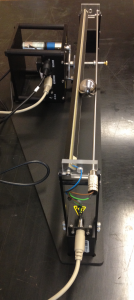10. This is an inverted pendulum (length is 33.7cm) which attaches to a rotary servo base unit (approximately 15cmX15cmX18cm) from Quanser.  To invert the pendulum requires stability theory and feedback control. The software this rotary unit uses is MathWork's Simulink. It was used in Math 3052 (Exploring Geometries) and MATH 4650 (Feedback Control Systems).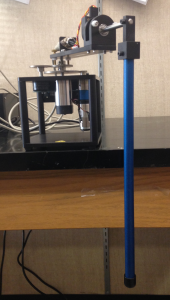9. This is a flexible link (length is 41.9cm) mounted on a rotary servo base unit (approximately 15cmX15cmX18cm) from Quanser.  It is often used to model vibrations in flexible structures, such as the wing of an airplane, and feedback control. It can also be used to model medical ailments such as tremors in limbs that arise as a symptom of a disease. The software this rotary unit uses is MathWork's Simulink. It was used in Math 3052 (Exploring Geometries) and MATH 4650 (Feedback Control Systems).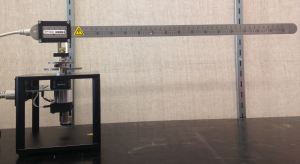8. This is a coupled tanks system (approximately 30.5cm X 30.5cm X 91.5cm) from Quanser. The software this coupled tanks system uses is MathWork's Simulink. It was used in Math 3052 (Exploring Geometries), MATH 3250 (Mathematical Biology), MATH 4090 (Mathematical Modelling) and MATH 4650 (Feedback Control Systems). See a video of the coupled tank in action. Credit: Professor Jane Heffernan.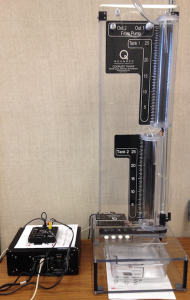7. This is a Lego Mindstorm EV3 (approximately 15cm X 19cm X 12cm), which is a programmable robot based on Lego building blocks. The corresponding software for these robots is available here. It was used in Math 3052 (Exploring Geometries), MATH 3250 (Mathematical Biology) and MATH 4090 (Mathematical Modelling). Credit: Gian Alix; Emily Di Bratto; Gina Faraj; Professor Jane Heffernan; Spencer Hooks; Professor Andrew Skelton.

See what other's are doing with the EV3:
http://www.stemcentric.com/ev3-tutorial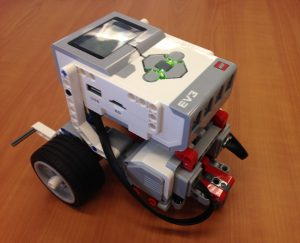6. This is a water tank (approximately 101.5cm x 15cm x 31cm) that generates waves by way of a compressed air generator. Instructions on how to use this can be found here. The study of waves explores mathematical concepts such as amplitude, sinusoidal functions, estimation, frequency, cycloids, etc. Credit: Kaloyan Bankov; Louis Conforti; Kevin Yu.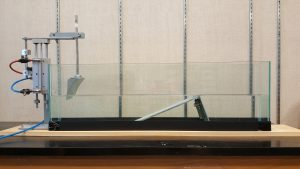5. This 3D printed piece (approximately 10cm x 10cm x 10cm) is a hypercube. It was used in Math 3052 (Exploring Geometries). Credit: Professor Andrew Skelton; ThingiverseShapeways.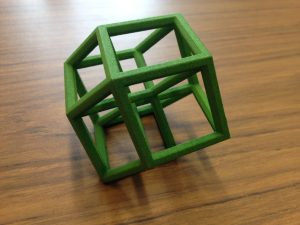4. This 3D printed "wobbly circle" piece (approximately 6cm x 12cm x 7cm) was obtained for teaching the idea of circles and how they move. It was used in Math 3052 (Exploring Geometries). The inspiration for this piece can be found here. Credit: Professor Andrew Skelton; ThingiverseShapeways.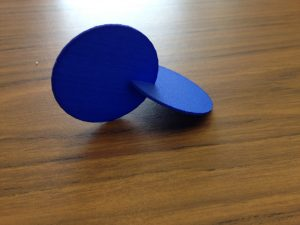3. This 3D printed piece (approximately 6cm x 7cm x 10cm) is a Klein Bottle. It was used in Math 3052 (Exploring Geometries) in F2018-W2019. Credit: Professor Andrew Skelton; ThingiverseShapeways.2. This 3D printed piece (approximately 8cm x 8cm x 10cm) is a Sierpinksi Pyramid. It was used in Math 3052 (Exploring Geometries). Credit: Professor Andrew Skelton; ThingiverseShapeways.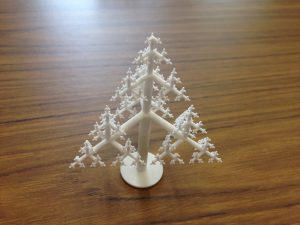1. This 3D printed piece (approximately 8cm x 10cm x 8cm) was obtained for teaching the idea of stereographic projections. It was used in Math 3052 (Exploring Geometries). Credit: Professor Andrew Skelton; ThingiverseShapeways.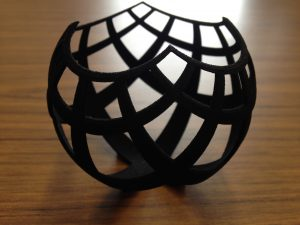This page was last updated on February 25, 2020; it was first created on September 11, 2018.